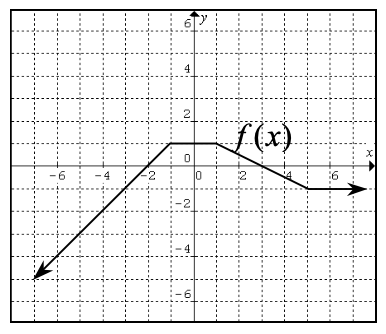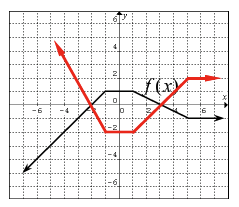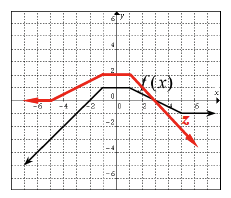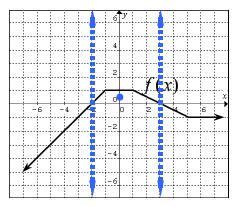### Home > PC > Chapter 7 > Lesson 7.1.2 > Problem7-30

7-30.

Using the graph of $f\left(x\right)$ at right, sketch the following functions.

1. $−2f\left(x\right)$

1. Reflect across the x-axis.
2. Stretch vertically by a factor of 2.

2. $f\left(−x\right) + 1$

1. Reflect across the y-axis.
2. Shift up 1 unit.

3. $\frac { 1 } { f ( x ) }$